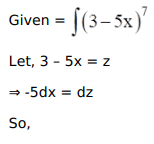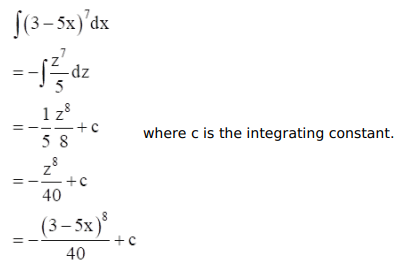# Mark against the correct answer in each of the following:

Question:

Mark $(\sqrt{ })$ against the correct answer in each of the following:

$\int(3-5 x)^{7} d x=?$

A. $-5(3-5 x)^{6}+C$

B. $\frac{(3-5 x)^{8}}{-40}+C$

C. $\frac{-5(3-5 x)^{8}}{8}+C$

D. none of these

Solution: